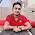# C Program to find Palindrome Number

Palindrome numbers are those which remains same after reversing. For a better concept I am quoting from Wikipedia:
palindromic number or numeral palindrome is a number that remains the same when its digits are reversed. Like 16461, for example, it is “symmetrical”. The term palindromic is derived from palindrome, which refers to a word (such as rotor or racecar) whose spelling is unchanged when its letters are reversed.
Here is a C Program to find Palindrome Number:

```#include <stdio.h>

int main()
{
int num, reverse=0, temp;
printf("Enter a number: ");
scanf("%d",&num);
temp = num;
while(temp != 0){
reverse = reverse * 10;
reverse = reverse + temp%10;
temp = temp / 10;
}
if(num==reverse) printf("The number is Palindrome");
else printf("The number in not Palindrome");
return 0;
}
```

Explanation of the program:
First we are taking three variables.  We are assigning the input into a variable temp. In the while loop actually we are reversing the input. Finally when we found that the given input and reversed number are equal then it will print The number is Palindrome.
Share:

#### 2 comments :

1.Palindrome Number Program in C++

A Palindrome number is a number that remains the same when its digits are reversed. Like 16461, for example: we take 121 and reverse it, after revers it is same as original.

2.Spam comments will be deleted. :)